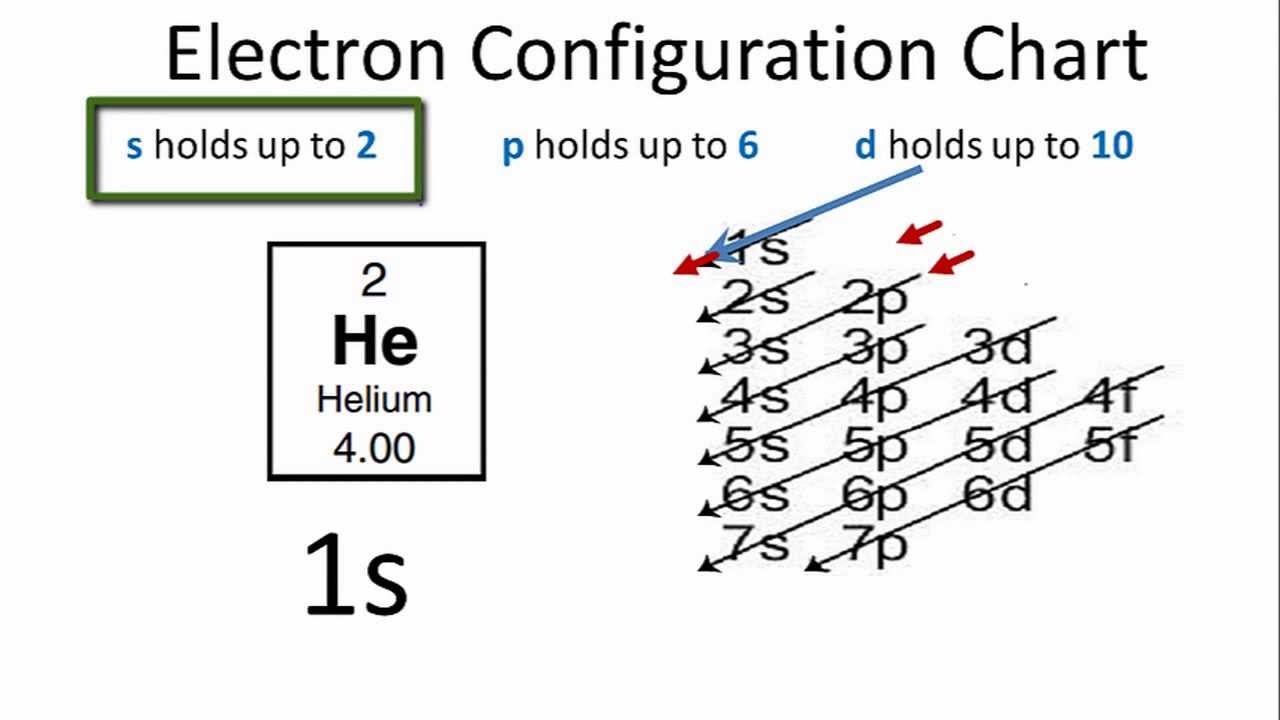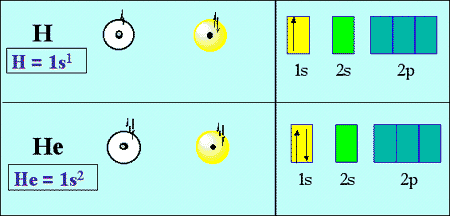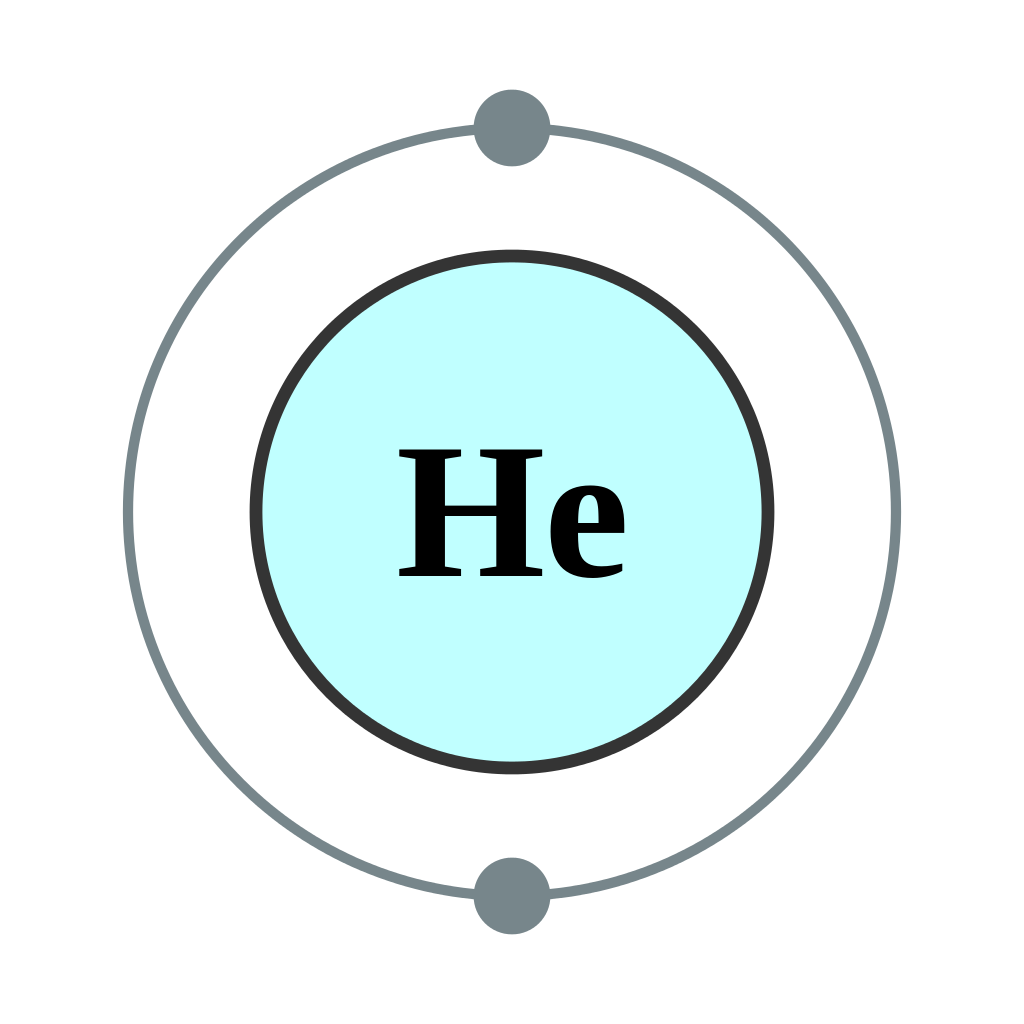# How To Find Electron Configuration For Helium {He}

In the periodic table, the second chemical element is Helium whose symbol is “He” and its atomic number is 2. Helium is the chemical element which is tasteless, colourless, odourless, inert, non-toxic, monoatomic gas and it lies in the noble gas group category. This element has a lowest boiling point.

As the electrons distribution on the orbit of particular atom/molecule is defined as the electron configuration of that atom or molecule. Similarly, helium has two electrons present at its first shell or orbit so the electronic configuration of helium is represented as:

## Electronic Configuration For Helium

1s2### Electron Configuration For Helium Ion

Helium has two electrons which are present at its first orbit that is 1s. So, the electron configuration for helium ion is 1s2.### Helium Number of Valence Electrons

Valence electrons are the number of electrons present at the outer most shell. Here the helium has only two electrons which are present in its one shell. So, the number of valence electrons for Helium is 2.

#### What is The Electron Configuration of Helium

Electron configuration of this noble gas element is 1s2 as helium consists of 2 electrons.#### How Many Valence Electrons are in helium

Helium has 2 valence electrons as the numbers of electrons which are in the outer orbit are considered as valence electrons and helium with 2 electrons is stable and the number of valence electrons is also 2 here.